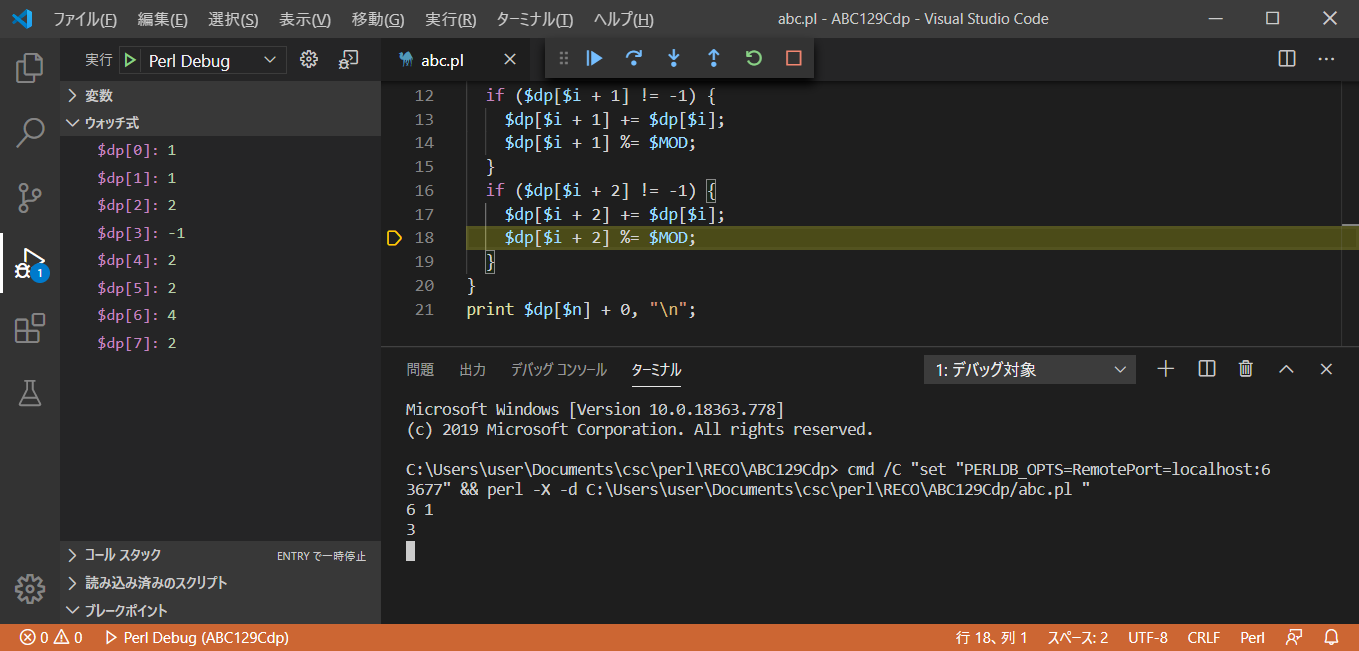# Introduction

• AtCoder Problems * Use Recommendations to solve past problems. Thanks to AtCoder and AtCoder Problems.

# This theme

AtCoder Beginner Contest 129 C - Typical Stairs Difficulty: 795

• Part 1 * The theme is Fibonacci The theme of the second part is dynamic programming Ruby Since the combination of the number of stairs was equal to the number of Fibonacci, the solution of the first part could be used, but is it something that can be solved more universally?

That's where the dynamic programming method found in * Official editorial * comes into play.

#### `ruby.rb`

``````
n, m = gets.split.map(&:to_i)
MOD = 1_000_000_007
dp = Array.new(n + 2, 0)
a = []
dp = 1
m.times do |i|
a[i] = gets.to_i
dp[a[i]] = -1
end
n.times do |i|
next if dp[i] == -1
if dp[i + 1] != -1
dp[i + 1] += dp[i]
dp[i + 1] %= MOD
end
if dp[i + 2] != -1
dp[i + 2] += dp[i]
dp[i + 2] %= MOD
end
end
puts dp[n]
``````

#### `array.rb`

``````
oks[a]=false;  # =>Broken floor array

dp[a[i]] = -1  # =>Broken floor-1
``````

The official editorial provides an array for broken floors, but for now, we'll embed it inside the DP array. In the watch type shown below, the **-1 ** part is the broken floor.When debugging with VSCode etc., the inside of the DP array is displayed in variables and watch expressions, so you can check how the numerical value changes and how to skip the broken floor.

#### `fib version.rb`

``````
if a[i] - a[i - 1] == 1
puts "0"
exit
end
``````

The source of the Dynamic Programming version does not have the part of the source of the Fibonacci version that determines whether the broken floor is continuous. No, but if there are consecutive broken floors, it properly returns ** 0 **.

In addition, it is easy to respond to changes in conditions such as being able to go up three steps.

Dynamic programming is amazing. Perl

#### `perl.pl`

``````
chomp (my (\$n, \$m) = split / /, <STDIN>);
my \$MOD = 1e9 + 7;
my (@a, @dp);
\$dp = 1;
for my \$i (1..\$m) {
chomp (my \$in = <STDIN>);
\$a[\$i] = \$in;
\$dp[\$a[\$i]] = -1;
}
for my \$i (0..\$n - 1) {
next if \$dp[\$i] == -1;
if (\$dp[\$i + 1] != -1) {
\$dp[\$i + 1] += \$dp[\$i];
\$dp[\$i + 1] %= \$MOD;
}
if (\$dp[\$i + 2] != -1) {
\$dp[\$i + 2] += \$dp[\$i];
\$dp[\$i + 2] %= \$MOD;
}
}
print \$dp[\$n] + 0, "\n";
``````
• Perl * takes advantage of the fact that if an undefined value is used in the calculation, it will be treated as ** 0 **. Java

#### `java.java`

``````
import java.util.*;

class Main {
public static void main(String[] args) {
Scanner sc = new Scanner(System.in);
int n = Integer.parseInt(sc.next());
int m = Integer.parseInt(sc.next());
int MOD = 1_000_000_007;
int a[] = new int[m];
int dp[] = new int[n + 2];
dp = 1;
for (int i = 0; i < m; i++) {
a[i] = Integer.parseInt(sc.next());
dp[a[i]] = -1;
}
sc.close();
for (int i = 0; i < n; i++) {
if (dp[i] == -1) {
continue;
}
if (dp[i + 1] != -1) {
dp[i + 1] += dp[i];
dp[i + 1] %= MOD;
}
if (dp[i + 2] != -1) {
dp[i + 2] += dp[i];
dp[i + 2] %= MOD;
}
}
System.out.print(dp[n]);
}
}
``````

This time it's addition, so it's AC </ font> without using long.

Ruby Perl Java
Code length 359 Byte 436 Byte 902 Byte
Execution time 64 ms 83 ms 347 ms
memory 3912 KB 12288 KB 44684 KB

# Summary

• ABC 129 C was solved with difficulty
• Become familiar with Ruby
• Become familiar with Perl
• Become familiar with Java
• Became familiar with dynamic programming

Referenced site